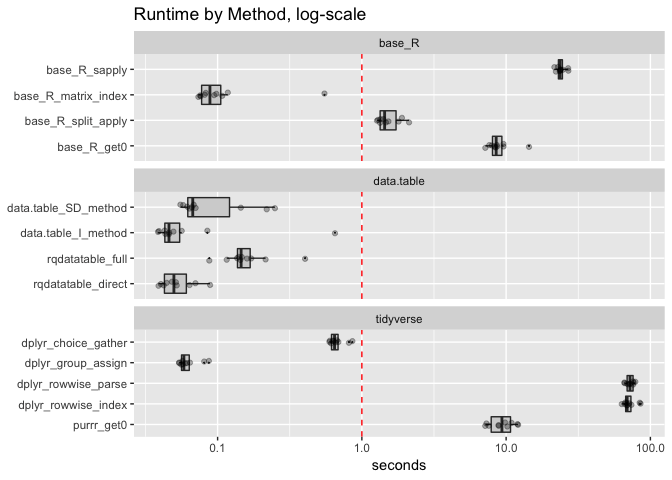# Timing Column Indexing in R

I’ve ended up (almost accidentally) collecting a number of different solutions to the “use a column to choose values from other columns in R” problem.

What we did is: build a 1,000,000 row variation of the original example. In this variation we ensured that the selections are all valid column names, and removed any extra code that the methods had included to defend against mis-matches. We then timed 13 solution variations. We present the results in a graph here.For this plot: being more to the left (having smaller run times) is better. We have marked 1 second as an important perceptual boundary (things longer than one second tend to be perceived as slow).

We have grouped the solution methods by the implementing family (base R, data.table, tidyverse). Each run is repeated 10 times in random order to try an get a good measurement. There are also several variations of solution strategy (matrix index, row-wise operation, and grouping by column choice to make the choice piecewise constant).

In this first graph we see that most methods run-times are under 1 second of run-time, “base_R_split_apply” is just about 1.5 seconds, and there is a remaining set of slow methods (typically taking between 9 to 72 seconds). All of the slow methods (base_R_sapply, base_R_get0, dplyr_rowwise_parse, dplyr_rowwise_index, purr_get0) are variations on user code running over rows. This confirms that running user code per-row is a usually an inefficient way to do things: even if you dress it up with an apply, map, rowwise, or so-on. One really wants to write vectorized code where the user code is written in terms of columns, and R itself (or a C/C++ package) is dealing with the rows.

The observed mean runtimes are given here.

```               method  mean_seconds
rqdatatable_direct   0.054
dplyr_group_assign   0.063
data.table_SD_method   0.106
data.table_I_method   0.110
base_R_matrix_index   0.136
rqdatatable_full   0.172
dplyr_choice_gather   0.676
base_R_split_apply   1.547
base_R_get0   9.038
purrr_get0   9.483
base_R_sapply  24.006
dplyr_rowwise_index  71.826
dplyr_rowwise_parse  72.227
```

Note: the fast timings have noise which makes ordering the fast methods by mean difficult. For instance rqdatatable_direct must be slower than data.table_I_method, as it essentially calls it. Also note: the data.table methods are paying the cost of copying/converting in their timings (which may not be entirely fair to data.table). Notice the means confuse method order, and the medians (portrayed on the graph) seem more reliable. Some of the variation in run times (and hence means) may be garbage collects (independent of method), which would require a large number of runs to average out of the fast measurements.

We can increase the legibility of the fast methods by switching to a log-scale plot.In this plot (which expands detail of the fast method timings at the expense of compressing detail of the slow method timings) we can see the data.table_I_method is routinely the fastest. This makes sense as this is an in-place indexing method designed to minimize data-motion. The rqdatatable timings are timings of rqdatatable using data.table to solve the problem in direct mode and base-R to solve the problem in full mode (it can also use data.table in full mode but we have not performed this timing which would fall between the two rqdatatable timings observed). Please keep in mind rqdatatable is not part of data.table, but a user of data.table.

Some notes on solution strategies:

• The dplyr_group_assign solution is a port of the data.table_SD_method to dplyr notation.
• The base_R_split_apply solution is also is a port of the data.table_SD_method to base-R methods.
• The base_R_matrix_index method is technique apparently unique to base-R (likely spending most of its time in a possibly avoidable match() sub-step).
• The dplyr_choice_gather method is interesting as it uses a data-reshaping (a tidyr::gather()). This may, at first, sound heavy-handed but it is in fact a very good idea. In fact in a database the data would have been stored in a thin/tall configuration (each fact in its own row, instead of multiple facts per row) and a join/filter based solution would be the natural solution.

Notice how solution strategy has a large influence on method speed. For example the splitting strategy is in general good, independent of package. Likewise row-wise strategies are bad, independent of package. Rowwise performance varies from pacakge to package, but avoiding user-rowwise code is a more important point.

Categories: Opinion Tutorials

Tagged as:### jmount

Data Scientist and trainer at Win Vector LLC. One of the authors of Practical Data Science with R.